Skip to content
Related Articles
Basic Operations in Octave
• Last Updated : 21 Jun, 2021

GNU Octave is a high-level programming language, primarily intended for numerical computations. It can also be used to implement various machine learning algorithms with ease. Octave is open-source i.e. it is free to use, whereas MATLAB is not thus MATLAB requires a licence to operate.
Below are the various basic functionalities of Octave :
1. Arithmetic Operations : Octave can be used to perform basic mathematical operations like addition, subtraction, multiplication, power operation etc.

## MATLAB

 `% addition operation``23 + 65 + 8` `% subtraction operation``32 - 74` `% power operation``6 ^ 2` `% multiplication operation``45 * 7` `% division operation``5 / 6`

Output :

```ans = 96
ans = -42
ans = 36
ans = 315
ans = 0.83333```

2. Logical Operations : Octave can be used to perform logical operations like AND, OR, NOT etc.

## MATLAB

 `% logical AND    ``1 && 0` `% logical OR``1 || 0` `% logical NOT``~1`

Output :

```ans = 0
ans = 1
ans = 0```

3. Relational Operations : Octave can be used to perform relational operations like greater than, less than etc.

## MATLAB

 `% equal to``1 == 1` `% not equal to``0 ~= 0` `% greater than   ``1 > 0` `% less than``1 < 0` `% greater than equal to ``1 >= 2` `% less than equal to``0 <= 0`

Output :

```ans = 1
ans = 0
ans = 1
ans = 0
ans = 0
ans = 1```

4. Changing the default Octave prompt symbol : The default Octave prompt symbol is “>>”. We can change the default Octave prompt symbol using the below commands :

## MATLAB

 `PS1(``'<< '``);``PS1(``'@ '``);``PS1(``'# '``);`

Output :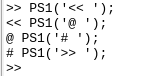5. Variables : Like other programming languages, Octave also has variables to temporarily store data.

## MATLAB

 `% variable declaration and initialization``var = 2` `% if we want to create the variable and don't want to print it``% then put a semicolon at the end of that command``var = 3; ``% this time the variable will not be printed` `% variable of datatype char``ch = ``'c'` `% storing the result of an operation in a variable``res = (1 != 1)` `% storing the value of pi in a variable``var = pi` `% printing a variable with disp() function``disp(var);` `% using sprintf() function to print a string``disp(sprintf(``'3 decimal values : %0.3f'``, var))` `% using format long to resize``format long``var` `% using format short to resize``format short``var`

Output :

```var =  2
ch = c
res = 0
var =  3.1416
3.1416
3 decimal values : 3.142
var =  3.141592653589793
var =  3.1416```

6. Matrices and Vectors : Now let’s learn how to deal with matrices and vectors in Octave. We can create matrix as shown below.

## MATLAB

 `% creating matrix in row major``matrix = [1 2 3; 4 5 6; 7 8 9]`

Output :

```matrix =

1   2   3
4   5   6
7   8   9```

We can also make a vector, a vector is a matrix with n rows and 1 column(column vector) or 1 rows with n columns(row vector). here in example 2 and 3 the middle value 5 and 0.5 shows that we want to make a vector matrix from range 1 to 20 with the jump of 5 and from range 0 to 5 with a jump of 0.5 respectively.

## MATLAB

 `% creating row vector``r_v = [1, 2, 3]` `% creating column vector``c_v = [1; 2; 3]`

Output :

```r_v =

1   2   3

c_v =

1
2
3```

Here are some utility shortcuts to create matrices and vectors :

## MATLAB

 `% creating vector using ":"``% the extreme end values denote the range``% and the middle value denotes the step``v1 = 1 : 5 : 20``v2 = 1 : 0.5 : 5` `% without the step parameter``v3 = 1 : 10` `% generate matrix of size 4x4 with all element as 1``ones_matrix = ones(4, 4)` `% generate matrix of size 4x4 with all element as 10``M = 10 * ones(4, 4)` `% generate row vector of size 5 with all elements 0``zeroes_vector = zeros(1, 5)` `% generate row vector of some random numbers between 0 and 1``random_vector = rand(1, 5)` `% generate matrix of some random numbers between 0 and 1``random_matrix = rand(3, 4)` `% generate matrix with Gaussian distribution``% where mean = 0 and variance and standard deviation = 1``gauss_matrix = randn(5, 5)` `% generate identity matrix with size 5x5``identity_matrix = eye(5)`

Output :

```v1 =

1    6   11   16

v2 =

1.0000    1.5000    2.0000    2.5000    3.0000    3.5000    4.0000    4.5000    5.0000

v3 =

1    2    3    4    5    6    7    8    9   10

ones_matrix =

1   1   1   1
1   1   1   1
1   1   1   1
1   1   1   1

M =

10   10   10   10
10   10   10   10
10   10   10   10
10   10   10   10

zeroes_vector =

0   0   0   0   0

random_vector =

0.79085   0.35395   0.92267   0.60234   0.75549

random_matrix =

0.64434   0.67677   0.54105   0.83149
0.70150   0.16149   0.38742   0.90442
0.60075   0.82273   0.37113   0.91496

gauss_matrix =

0.705921   1.336101  -0.097530   0.498245   1.125928
-0.550047  -1.868716  -0.977788   0.319715  -0.603599
-0.018352  -2.133200   0.462272   0.169707   1.733255
0.623343   0.338734   0.618943   1.110172   1.731495
-1.741052  -0.463446   0.556348   1.633956  -1.424136

identity_matrix =

Diagonal Matrix

1   0   0   0   0
0   1   0   0   0
0   0   1   0   0
0   0   0   1   0
0   0   0   0   1```

7. Histograms : We can draw the histograms hist() function. We can also change the bucket size or bins of the histogram.

## MATLAB

 `% generate a vector with 1000 elements``elements_1000 = 1 + sqrt(25)*(randn(1, 1000));` `hist(elements_1000 )`

Output :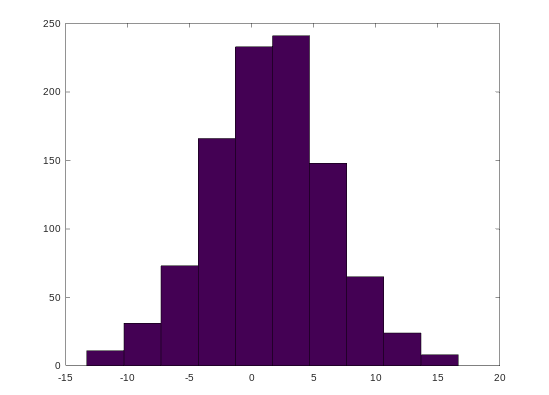## MATLAB

 `% generate a vector with 1000 elements``elements_1000 = 1 + sqrt(25)*(randn(1, 1000));` `% histogram with 30 bins``hist(elements_1000, 30)`

Output :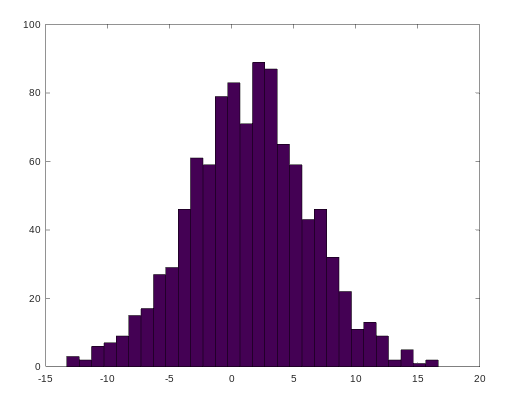8. Help : We can use the help command to see the documentation for any function.

## MATLAB

 `help eye``help sqrt``help hist`

Output :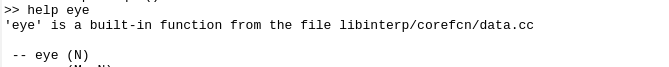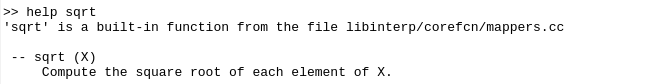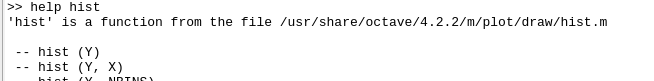My Personal Notes arrow_drop_up